Transforming Exponential Functions
 Home > Lessons > Transforming Exp Functions Search | Updated April 15th, 2021
Introduction

This lesson will inform you how to transform exponential functions. Here are the sections within this lesson page:

 Rules of Transformations Transformations -- regardless of the function -- behave the same. This graphic organizer describes transformations on the function f(x).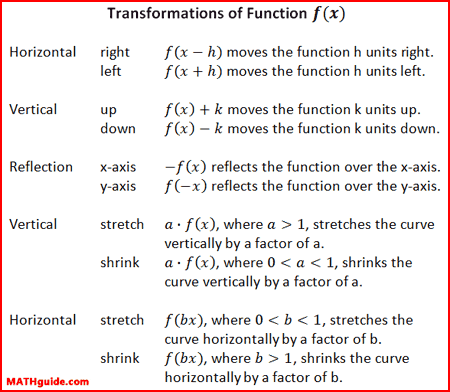The sections below will describe how specifically an exponential function behaves under these transformations. Horizontal Shifts and the Y-intercept If the x-variable of a parent function, f(x), is replaced with 'x + 2,' every point of the function will move 2 units left. Conversely, if the x-variable of a parent function, f(x), is replaced with 'x - 2,' every point of the function will move 2 units right.     The graph below demonstrates this for the function...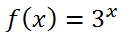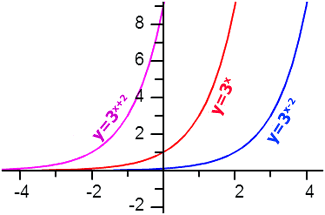There are two important points to notice. Point 1: The asymptotes for the three functions are all the same. All of the asymptotes are y = 0 because horizontal shifts do not move horizontal lines. The asymptotes for exponential functions are always horizontal lines. Point 2: The y-intercepts are different for the curves. Finding the location of a y-intercept for an exponential function requires a little work (shown below).     To determine the y-intercept of an exponential function, simply substitute zero for the x-value in the function. The reason this works is because all points on the y-axis have an x-value equal to zero.     Here is the mathematics for all three of the functions that have been graphed above. Colors have been added to match the graph in this section.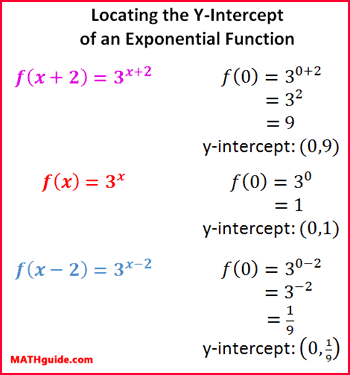These y-intercepts can be verified by examining the graphs in this section.uiz: Exponential Functions: Transformations Vertical Shifts and the Asymptote The parent curve of an exponential function has the asymptote y = 0. This is evident by looking at the function......which has been graphed below.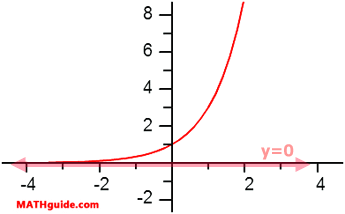Look what happens when we either add or subtract a number to/from our parent function.Notice if we add the number 1 to the function that the function moves vertically up 1 unit. If we subtract 1 to the function, the function moves vertically down 1 unit. In both cases the asymptote follows the curve. The table below shows this close correlation.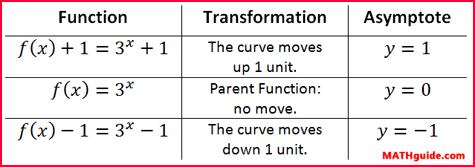uiz: Exponential Functions: Transformations Reflection Over X-Axis If a function and its opposite are compared, we would see that they would be mirror versions of each other. The mirror would be the x-axis, in this situation.     We should look at a specific situation. Let us examine our parent function from a previous section and its opposite function.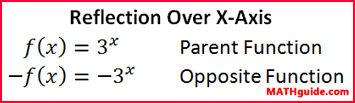Here are their graphs.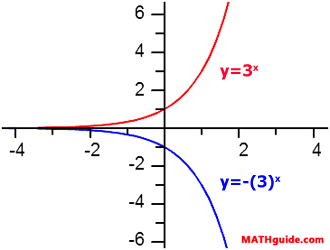This demonstrates how the transformed function is obtained by flipping the original function over the x-axis. Transformations: Examples Now that we have seen several types of transformations, we will now look at a few examples. Example 1: g(x) = 4x+2 + 1     Let's first determine how this function compares with its parent function, which is...To graph g(x), we would have to move h(x) 2 units left and 1 unit up.     The number next to the x-value is the horizontal shift and we have to take the opposite to determine the direction of the shift. The +2 really means 2 units left. The +1 is not next to the x-value, which means it is the vertical shift number.     Now, we can sketch the graph of g(x) since we have a general idea of the shape of h(x), which is an exponential growth function.     The asymptote of h(x), which is y = 0, will shift up 1 unit along with g(x). This will make the asymptote of g(x) equal to y = 1.To locate its y-intercept, we need to substitute the value 0 for the x-value, like so.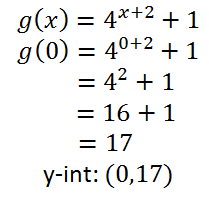Example 2: k(x) = -2x-1 - 3     This transformation requires reflecting k(x) over the x-axis, moving the curve 1 unit right and 3 units down.     The asymptote must be y = -3, since the curve was moved down 3 units.     The y-intercept can be found as follows.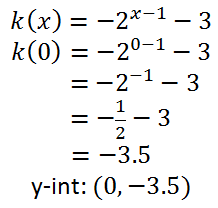uiz: Exponential Functions: Transformations Instructional Videos This video will help you understand the transformation sections above.ideo: Basic Translations (Transformations) of Functions Interactive Quizzes This quiz will help you master the lessons above.uiz: Exponential Functions: Transformations Related Lessons Try these lessons, which are related to the sections above.esson: Translationsesson: Translating Polynomials: Parabolasesson: Exponential Functionsesson: Calculating Value Over Timeesson: Geometric Sequences and Seriesesson: Logarithms